## research papersJOURNAL OFSYNCHROTRONRADIATION
ISSN: 1600-5775
Volume 26| Part 4| July 2019| Pages 1192-1197

## A variable fixed-focus constant optimization method for a variable-included-angle varied-line-spacing plane-grating monochromator

aShanghai Advanced Research Institute, Chinese Academy of Sciences, Shanghai 201210, People's Republic of China, bShanghai Institute of Applied Physics, Chinese Academy of Sciences, Shanghai 201800, People's Republic of China, and cShanghai Synchrotron Radiation Facility, Shanghai 201204, People's Republic of China
*Correspondence e-mail: wuyanqing@sinap.ac.cn

(Received 30 January 2019; accepted 3 April 2019; online 4 June 2019)

Variable-included-angle varied-line-spacing plane-grating monochromators (VIA-VPGM) have been applied to many beamlines because of the self-focusing and aberration-correction function of the varied-line-spacing grating. Unfortunately, to optimize the variable-line-spacing coefficient of the grating, the fixed-focus constant (Cff) has to be fixed first in the VIA-VPGM. In this way, some of the advantages of these monochromators are lost, such as the flexibility of choosing a different energy-resolving power by varying the Cff. In this work, a variable Cff optimization method is introduced for a VIA-VPGM. By adopting this method, the Cff could be arbitrarily selected in the VIA-VPGM.

### 1. Introduction

At a synchrotron radiation or free-electron laser facility, a monochromator is used to transmit a mechanically selectable narrow band of wavelengths of radiation chosen from a wider range of wavelengths available at the input. It is almost the most important device in the beamline. Among the various kinds of soft X-ray monochromator, the variable-included-angle plane-grating monochromator (VIA-PGM) (Petersen, 1982; Riemer & Torge, 1983; Pimpale et al., 1991; Follath & Senf, 1997) is one of the most successfully developed optics of the last few decades. There are numerous advantages of this type of monochromator. Besides covering a wide energy range, the VIA-PGM can also be operated in different modes depending on experimental need, such as a high-energy-resolution mode, a higher-order harmonic suppression mode and a high-flux mode, by selecting a suitable value of the fixed-focus constant, Cff. Based on these advantages, VIA-PGMs have been employed on many synchrotron radiation beamlines all over the world (Xue et al., 2010; Aksela et al., 1994; Follath, 2001; Warwick et al., 2001; Follath et al., 1998).

Usually, in the VIA-PGM setup, a pre-collimating mirror and a re-focusing mirror are necessary because a parallel incident beam is required by the grating, which is unable to focus the beam. Unfortunately, additional aberrations will be introduced by these focusing mirrors, which is detrimental to improving the energy resolution of the beamline. To overcome this shortcoming, and with the development of the grating manufacturing process, a variable-line-spacing (VLS) plane grating is now applied in some newly designed VIA-PGMs called VIA-VPGM (Xue et al., 2014; Reininger & de Castro, 2005; Ono et al., 2004; Amemiya et al., 2010), which is a significant improvement on the original design. Because of its self-focusing and aberration-correction function, the beam can be focused at the exit slit using a VLS grating, without introducing coma and spherical aberrations into the system.

Although the performance of a beamline can be improved by applying the VIA-VPGM, this type of monochromator can no longer be operated in a flexibly operating mode. This is mainly because the Cff value has to be fixed at a certain reference energy at the start of the design process for a monochromator. The variable-line-spacing coefficient of the VLS grating can be obtained only after determining the Cff, thereby achieving focusing and aberration correction. In order to focus X-ray beams of different wavelengths onto the same focus, the Cff will vary slightly with wavelength. However, each wavelength corresponds to a fixed C value, otherwise the focus condition cannot be met. It is precisely because the Cff cannot be arbitrarily varied in the VIV-VPGM that this monochromator can be operated in only one mode.

In this work, a variable Cff optimization method is introduced for the VIA-VPGM. By adopting this method, the Cff can be arbitrarily selected. The VIA-VPGM can then also be operated in flexible operating modes. It provides a possibility of implementing more operating modes using fewer gratings in practical applications.

### 2. Optimization method

For a VLS grating, the line spacing d is a function of the position w in the dispersive direction. The function can be expanded as a power series in w, namely (McKinney, 1992)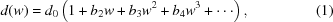where d0 is the line spacing at the center of the grating, and b2b3 and b4 are the space-variation parameters. The defocus term (F20) and the coma term (F30) in an optical path function can be eliminated by choosing an appropriate linear coefficient term b2 and quadratic term b3, respectively, according to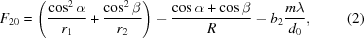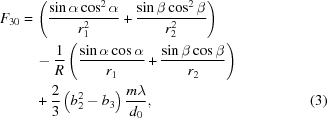where m is the diffraction order, α is the incidence angle, β is the diffraction angle, r1 is the objective distance, r2 is the imaging distance and R is the grating radius (for a plane grating, R → ∞). When F20 = 0, the focusing condition is satisfied. For a plane VLS grating,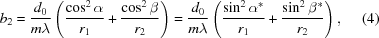where α* is the grazing incidence angle and β* is the complementary angle of diffraction. If r1 = −r2,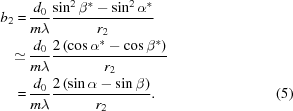Here, the approximation cos x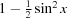is used (Sinn et al., 2012) since usually both α* and β* are very small in an actual grating monochromator. The error in the above approximation will be minimal when both the α* and β* angles are very small. Considering the grating equation sin α − sin β = mλ/d0, we can obtain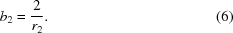Therefore, when the imaging distance is equal to the virtual object distance, the focusing condition of a VLS grating can be independent of other factors besides the imaging distance.

An optical schematic diagram of the new optimized VIA-VPGM is shown in Fig. 1. In this optimization method, a pre-focus mirror is necessary to produce a converging beam and a virtual source behind the grating to meet the precondition of r1 = −r2. The grating then produces a real image on the exit slit, and the exit slit is the real focus of the pre-mirror. In order to illustrate the improved performance of this new optimization method, we take a 4 m-long undulator as a realistic optical source and typical distance values between optical elements for a model VIA-VPGM as an example. The beam sizes and divergences are calculated from the vector sum of the electron beam root-mean-square (r.m.s.) values on the orbit (σx,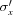, σy,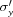) and the radiation values (σr,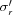). The radiation values are calculated using the approximations σr =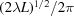and=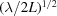, where λ is the wavelength of the radiation and L is the length of the insertion device. For the model monochromator, the object distance of the pre-focusing mirror is 22 m and the imaging distance of the grating is 18 m. These two optical elements are separated by 5 m. Detailed parameters for the model monochromator are given in Table 1.

 Table 1Detailed parameters for the model monochromator
 Electron beam r.m.s. size Horizontal σx (mm) 0.15855 Vertical σy (mm) 0.00987 Electron beam r.m.s. divergence Horizontal(rad) 3.2914 × 10−5 Vertical(rad) 3.9497 × 10−6 Undulator length (mm) 4000 Pre-focusing mirror sagittal slope error (rad) 2 × 10−6 Object distance of the pre-focusing mirror (mm) 22000 Incident angle of the pre-focusing mirror (rad) 0.02 Distance from the source to the grating(mm) 27000 Grating slope error (rad) 1 × 10−7 Plane mirror slope error (rad) 1 × 10−7 Imaging distance of the grating (mm) 18000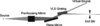Figure 1 An optical schematic diagram of the new optimized VIA-VPGM.

The energy range covered by the model monochromator is chosen as 200–2000 eV and the grating line density is assumed to be 1000 lines mm−1. By adopting this new optimization method, the linear coefficient term b2 in equation (2)can be calculated according to equation (6), while the quadratic term b3 has to be optimized at a selected reference energy and Cff value. In the model monochromator, the coma aberration is eliminated at 1000 eV with Cff = 3. A few parameters can be derived under these conditions and are listed in Table 2.

 Table 2Derived parameters for the model monochromator
 Virtual source of the grating (mm) −18000 Sagittal curvature radius of the pre-focusing mirror (mm) 449.75 b2 (mm−1) 1.11111 × 10−4 b3 (mm−2) 3.04628 × 10−9

The energy-resolving power (RP) calculated in this study is mainly determined by seven factors: source size, exit slit size, meridian slope error of the grating and focusing mirror, aberrations from defocus and coma, and grating diffraction limit. High-order aberrations (smaller than F30) are small and negligible. Fig. 2shows the calculated RP of the model monochromator at various Cff values, with the RP defined as the inverse of the relative spectrum width (RSW). Their contributions to the relative spectrum width (RSW) Δλ/λtotal are as follows: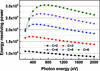Figure 2 The calculated RP at various Cff values for the model monochromator, with the exit slit size taken as the FWHM of the focused beam spot in the dispersion direction.

Source size: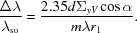Exit slit size: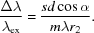Meridian slope errors of grating: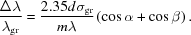Meridian slope errors of mirror: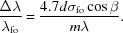Aberration from defocus: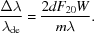Aberration from coma: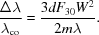Diffraction limit: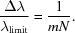Thus,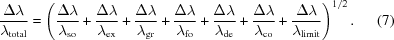Here, ΣyV is the r.m.s. value of the virtual source size (ΣyV = MΣyA, M is the magnification of the pre-focusing mirror and ΣyA is the r.m.s. value of the actual source size), σgr and σfo are meridian r.m.s. slope errors of the grating and the focusing mirror, s is the exit slit size, W is the half-width of the ruled area of the grating and N is number of coherently illuminated grating grooves. To guarantee sufficient photon flux, the exit slit size is taken as the FWHM of the focused beam spot in the dispersion direction.

By adopting this optimization method, the maximum Cff is decided by the footprint of the photon beam on the grating and the effective length of the grating. If the effective length of the grating is limited to 120 mm in this model monochromator, the maximum Cff values can be up to 6. It can be seen from Fig. 2that the RP increases as Cff increases. This trend is the same as for a traditional VIA-PGM. Therefore, a Cff value can be chosen flexibly according to actual need in the VIA-VPGM. The shadow ray-tracing results (Fig. 3) are consistent with the calculated RP results.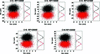Figure 3 Ray-tracing results for the model monochromator at 1000 eV at various Cff values.

### 3. Discussion

#### 3.1. Defocus aberration

Although the linear coefficient term b2 can be obtained according to equation (6)in the optimization method, an approximation has been used in this process which will introduce defocus aberration. The introduced defocus aberration will reduce the energy resolution, as shown at the low-energy end of Fig. 2. This is mainly caused by two factors. One is that the footprint of the photon beam on the grating becomes larger as Cff increases, which will also increase the defocus aberration at the same time. The other is that the RSW contributed by other factors, such as source size and exit slit, will decrease as Cff increases. Finally, the largest contribution to the RSW will be from the defocus aberration when Cff is large, to a certain extent. Therefore, to reduce the effect of defocus aberration, it is better to obtain a more accurate b2. If an approximation is used in equation (5), then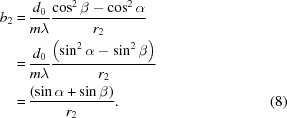Accurate b2 values calculated at various Cff values for the model monochromator are shown in Fig. 4, and the accurate (sin α + sin β) values corresponding to the b2 values are listed on the right of the plot. As mentioned above, the error of the approximation used in equation (5)will be minimal when both the incidence and diffraction angles are very large. As shown in Fig. 4, the value of sin α + sin β is closer to 2 when the monochromator is operated at the high photon energy end or with higher Cff values. Thus, the RP of the monochromator is not affected by the defocus aberration at the high photon energy end, while it is greatly affected by the defocus aberration at the low photon energy end. Therefore, to ensure optimal monochromator performance, the appropriate b2 value can be selected according to the value of sin α + sin β in different photon energy ranges. This is more in line with the real monochromator optimization process. The energy range in the model monochromator is out of the coverage capability of a single grating in actual use, since it is hard to maintain the diffraction efficiency of a single grating at a high level over such a wide range. Fig. 5shows the calculated RP with various Cff values of the model monochromator by choosing b2 = 1.995/r2 for an energy range of 200–800 eV and b2 = 1.999/r2 for an energy range of 800–2000 eV.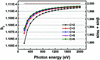Figure 4 Accurate b2 values calculated at various Cff values for the model monochromator, and the accurate (sin α + sin β) value corresponding to the b2 value.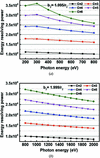Figure 5 The calculated RP at various Cff values for the model monochromator by choosing (a) b2 = 1.995/r2 for the energy range 200–800 eV and (b) b2 = 1.999/r2 for the energy range 800–2000 eV.

The discussion above is based on the case of fixed exit slit, which is beneficial for re-focusing the beam at the endstation. If a movable exit slit is available, the defocus aberration can be eliminated perfectly by moving the exit slit a few centimetres.

#### 3.2. Coma aberration

The quadratic term b3 has to be optimized at a reference energy with a selected Cff. In this study, the coma aberration is also optimized at 1000 eV with a Cff of 3. Similar to the case of defocus aberration, the coma aberration will increase as Cff increases because of its larger footprint on the grating, no matter what Cff value is chosen for optimization. Although the coma aberration is no longer eliminated at the reference energy when Cff varies, the contribution to the RSW from the coma aberration is still negligible, as shown in Fig. 6.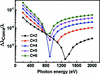Figure 6 Contribution to the RSW from the coma aberration at various Cff values for the model monochromator.

#### 3.3. Angle correction

In a synchrotron radiation facility, the grating is usually placed horizontally for a higher RP because the source size is smaller vertically than horizontally. In the model monochromator, the first focusing mirror is placed vertically, so the thermal deformation of this mirror has less effect on the RP while the horizontal spot is affected. The main contribution to RP degradation is from the deformation of the first plane mirror in the monochromator due to the heat load. This deformation can be equivalent to the range of the grating's virtual object distance. In practice, the pre-focusing mirror radius RF and the grating coefficient b2 have machining errors, and the mirrors' shape would also be deformed by the absorbed heat load. These will decrease the RP of the designed monochromator. For the VLS grating, to satisfy equation (6), the error in the b2 coefficient could be better compensated by adjusting the image distance accordingly, based on the measured precise b2 value. For the pre-focusing mirror, both the manufacturing error in the mirror radius and the deformation caused by the heat load will lead to a change in the grating's virtual object distance. Nevertheless, such effects can be corrected by properly adjusting the incident angle of the pre-focusing mirror. Introducing errors of 20% on the virtual object distance, the ray-tracing results before and after angle correction at 1000 eV with Cff = 3 are shown in Fig. 7. A decrease in RP can be seen when the errors are introduced in the model monochromator, while the final RP is almost the same as for the original design by adjusting the incident angle by 1.5 mrad. However, this angle adjustment would cause a displacement of the focusing spot at the exit slit. Therefore, one more mirror may be needed after the monochromator to re-correct the beam direction, which would lead to a slight loss of photon flux due to reflectivity limitations. So, the ultimate resolving power is not sacrificed, thanks to the angle correction, while the trade-off is that the photon flux may be reduced slightly because of the potential beam-direction re-correcting mirror after the monochromator.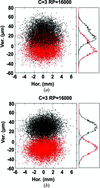Figure 7 Ray-tracing results for the model monochromator at 1000 eV at various Cff = 3, considering errors of 20% on the virtual object distance, (a) before and (b) after angle correction.

### 4. Conclusions

In this study, a new optimization method is given for optimizing variable-included-angle varied-line-spacing plane-grating monochromators (VIA-VPGMs). By adopting this method, the linear coefficient term b2 of the VLS grating is independent of Cff and only related to the image distance of the grating. Therefore, the Cff can be arbitrarily selected in the VIA-VPGM and the monochromator can be operated in flexible operating modes, the same as the traditional variable-included-angle plane-grating monochromator. Ray-tracing results demonstrate the energy-resolving power of the monochromator at different Cff values.

### Acknowledgements

The authors wish to thank the staff of the BL08U beamline at the SSRF.

### Funding information

The following funding is acknowledged: National Key R&D Program of China (grant Nos. 2017YFA0206001 and 2016YFA0401302); Youth Innovation Promotion Association of the Chinese Academy of Sciences (grant No. 2017306); National Natural Science Foundation of China (grant Nos. 11875314, U1632268 and 51705369); Shanghai Science and Technology Commission Research Projects (grant No. 17JC1400802); the State Key Laboratory of Applied Optics (China).

### ReferencesJOURNAL OFSYNCHROTRONRADIATION